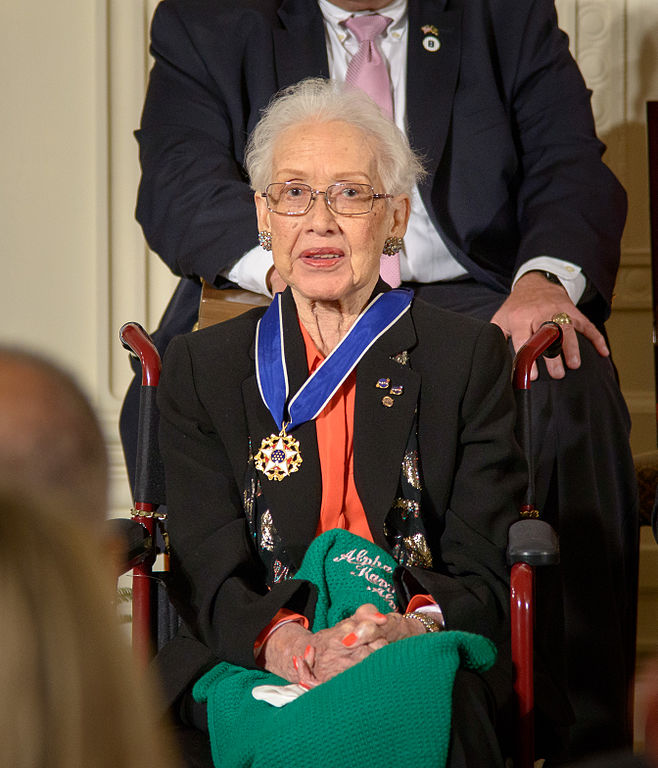# Blog

Viewing posts from February, 2018

### Thinking Out Loud – EGMO 2017 Problem 1

EGMO 2017, Problem 1. Let $$ABCD$$ be a convex quadrilateral with $$\widehat{DAB} = \widehat{BCD} = 90^\circ$$ and $$\widehat{ABC} > \widehat{CDA}$$. Let $$Q$$ and $$R$$ be points on segments $$BC$$ and $$CD$$, respectively, such that line $$QR$$ intersects lines $$AB$$ and $$AD$$ at points $$P$$ and $$S$$, respectively. It is given that $$PQ = RS$$. Let the midpoint of $$BD$$ be $$M$$ and the midpoint of $$QR$$ be $$N$$. Prove that the points $$M, N, A$$ and $$C$$ lie on a circle.

### Women in Mathematics beyond Stereotypes – Katherine JohnsonAnother mathematician for our series, one of whom is said “NASA would not be what it is if not for Katherine Johnson”

Un'altra matematica per la nostra serie, una di cui si è detto “La NASA non sarebbe ciò che è se non fosse stato per Katherine Johnson”

Tags:

### Thinking Out Loud – EGMO 2015 Problem 4

EGMO 2015, Problem 4. Determine whether there exists an infinite sequence $$a_1, a_2, a_3, \dots$$ of positive integers which satisfies the equality $a_{n+2}=a_{n+1}+\sqrt{a_{n+1}+a_{n}}$ for every positive integer $$n$$.

### EGMO Countries – AustriaThis is the second entry in a series of posts about the teams taking part in EGMO 2018. Today we have an interview with Birgit Vera Schmidt, the austrian Team Leader.

Questo è il secondo di una serie di post che ci faranno scoprire qualcosa di più sulle squadre presenti alle EGMO 2018. Oggi intervistiamo Birgit Vera Schmidt, la Team Leader austriaca.

Tags:

### Thinking Out Loud – EGMO 2014 Problem 3

EGMO 2014, Problem 3. We denote the number of positive divisors of a positive integer $$m$$ by $$d(m)$$ and the number of distinct prime divisors of $$m$$ by $$\omega(m)$$. Let $$k$$ be a positive integer. Prove that there exist infinitely many positive integers $$n$$ such that $$\omega(n) = k$$ and $$d(n)$$ does not divide $$d(a^2+b^2)$$ for any positive integers $$a, b$$ satisfying $$a + b = n$$.